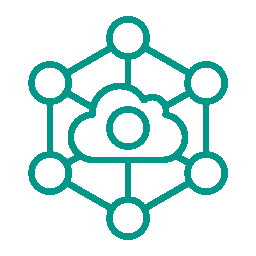•• ARTICLE
• STRING
• CONVERTER
• ENCRYPT
• NETWORK
• MORE
CHART
MATH
COORDINATE
IMAGE
• ARTICLE
STRING
CONVERTER
ENCRYPT
NETWORK
MORE
CHART
MATH
COORDINATE
IMAGEOnline Tools

# True Code/One's Complement/Two's Complement Calculator Online

TAG hardware

True Code/One's Complement/Two's Complement Calculator Online-summary

Online true code, One's Complement code, and Two's Complement calculator, supporting the calculation of 8 bit, 16 bit, 32 bit, 64 bit, and any bits of integer number's true code, One's Complement code, and Two's Complement.

True Code/One's Complement/Two's Complement Calculator Online-instructions
1. True code representation : The highest bit is the sign bit, where 1 represents a negative number and 0 represents a positive number. The remaining bits represent numerical values.
2. One's Complement representation : The One's Complement of a positive number is itself, while the One's Complement of a negative number is based on the true code, with the sign bit unchanged and the remaining bits taken as the inverse.
3. Two's Complement representation : The Two's Complement of a positive number is itself, while the Two's Complement of a negative number is based on the true code, with the sign bit unchanged and the other bits taken as inverse, and then +1 (i.e.+1 on the basis of the One's Complement).
4. Bits : The binary bits of an integer, can be selected or customized. When customizing, the range of bits is [2,1000000] 。 The range of values that can be entered as integers is [-2^(n-1) , 2^(n-1) - 1] ,Where n is the number of bits.
5. Integer : Enter an integer and calculate its true code, one's complement, and two's complement.